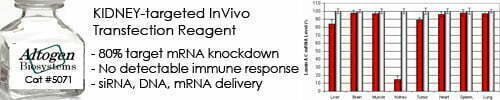••••Shop Products

# Molarity Calculator

A molarity calculator is a tool that calculates the molarity of a solution based on the amount of solute and volume of solvent used. Molarity is a measure of the concentration of a solution and is defined as the number of moles of solute per liter of solution.

The formula for calculating molarity is:

Molarity = moles of solute / volume of solution in liters

Where:

• Moles of solute is the amount of solute used, usually measured in moles (mol)
• Volume of solution is the total volume of the solution, usually measured in liters (L)

To use a molarity calculator, you need to input the values of moles of solute and volume of solution, and the calculator will calculate the corresponding molarity for you. The resulting molarity value can then be used to determine the concentration of the solution and to calculate other properties such as osmolarity, pH, and reaction rates.

Molarity is a commonly used unit in chemistry, biochemistry, and other related fields, and it is used to express the concentration of solutions in a standardized way that is easy to compare and manipulate.

Molarity Calculator
Enter formula weight:
g/mol
Desired final volume:
liters (L)
Desired concentration:
molar (M)
Desired concentration:

Sorry, comments are closed for this post.